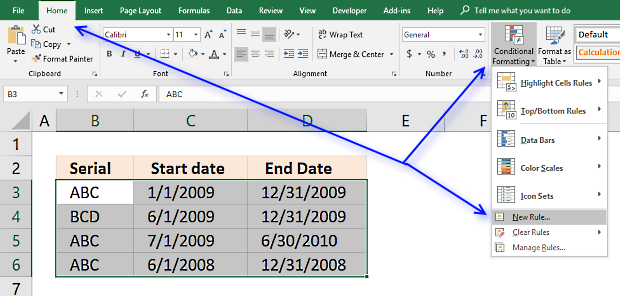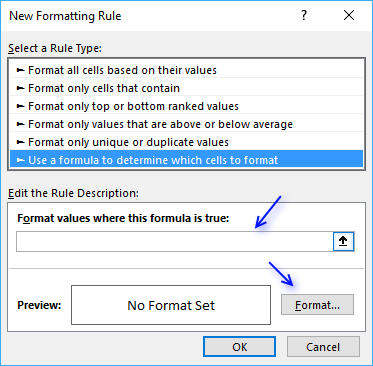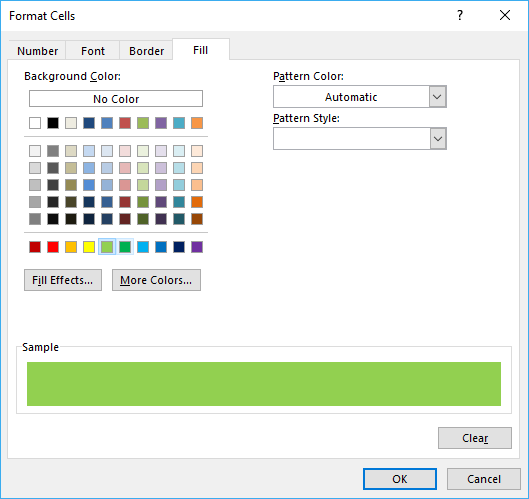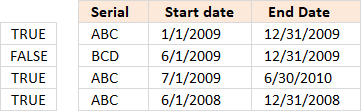Author: Oscar Cronquist Article last updated on October 28, 2018

Hi, I have a situation where I want to count if this value is duplicate and if it the dates are overlapping as well. Is there anyway to work this out?

eg
Serial | Start date | End Date
ABC | 01.01.2009 | 31.12.2009
BCD | 01.06.2009 | 31.12.2009
ABC | 01.07.2009 | 30.06.2010

So it should list the first and third entry.

The image above demonstrates a conditional formatting formula that highlights records based on a condition (column B) and date ranges (column C and D).

Records on row 3 and 5 are highlighted, they share the same condition in column B and their date ranges overlap.

#### How to apply conditional formatting formula to the data set

1. Select a cell range
2. Click the "Home" tab
3. Click "Conditional Formatting" button
4. Click "New Rule.."5. Click "Use a formula to determine which cells to format"
6. Type =SUMPRODUCT((\$C3<=\$D\$3:\$D\$6)*(\$D3>=\$C\$3:\$C\$6)*(\$B3=\$B\$3:\$B\$6))>1 in "Format values where this formula is TRUE" window.7. Click "Format.." button
8. Click "Fill" tab
9. Select a color for highlighting cells.10. Click "Ok"
11. Click "Ok"
12. Click "Ok"

### Explaining CF formula in cell B3

The formula is a conditional formatting formula, however, you can enter it in cell E3. Then copy the cell and paste to cells below.

Select cell E3 and then start the "Evaluate Formula" tool on the "Formula" tab on the ribbon.

Click "Evaluate" button to go through each calculation step.

#### Step 1 - Check if cells are equal to cell value

The equal sign lets you compare a cell value with a range. If it is the same the logical expression returns TRUE, if not FALSE.\$B3 is locked to column B, however, the row number changes as the CF formula is applied to cells below.

\$B3=\$B\$3:\$B\$6

becomes

"ABC "={"ABC ";"BCD ";"ABC ";"ABC "}

and returns {TRUE;FALSE;TRUE;TRUE}.

#### Step 2 - Check if date ranges overlap

The following two logical expressions check which date ranges overlap with the first record. The parenthes make sure that the order of calculation is correct. We want the comparisons to be performed first and then multiply the arrays.

(\$C3<=\$D\$3:\$D\$6)*(\$D3>=\$C\$3:\$C\$6)

becomes

(39814<={40178;40178;40359;39813})* (40178>={39814;39965;39995;39600})

{TRUE;TRUE;TRUE;FALSE}* {TRUE;TRUE;TRUE;TRUE}

and returns {1;1;1;0}

#### Step 3 - Multiply arrays

(\$C3<=\$D\$3:\$D\$6)*(\$D3>=\$C\$3:\$C\$6)*(\$B3=\$B\$3:\$B\$6)

{TRUE;FALSE;TRUE;TRUE}* {1;1;1;0}

and returns {1;0;1;0}

#### Step 4 - Sum values in array

SUMPRODUCT((\$C3<=\$D\$3:\$D\$6)*(\$D3>=\$C\$3:\$C\$6)*(\$B3=\$B\$3:\$B\$6))

becomes

SUMPRODUCT({1;0;1;0})

and returns 2.

#### Step 5 - Check if sum is larger than 1

SUMPRODUCT((\$C3<=\$D\$3:\$D\$6)*(\$D3>=\$C\$3:\$C\$6)*(\$B3=\$B\$3:\$B\$6))>1

becomes

2>1

and returns TRUE. Cell B3 is highlighted.